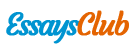# Chemistry Holt McDougal Chapter 2 Notes

Autor:   •  February 6, 2018  •  1,998 Words (8 Pages)  •  173 Views

Page 1 of 8

...

-Devices used for measuring mass in a lab are called balances. Balances can be electronic or mechanical, such as a triple-beam bass.

-The mass of the object never changes, no matter its location. However, the weight of the object is affected by its location so it changes. The weight depends on the gravity while the mass doesn’t.

-Weight – The force produced by gravity acting on mass.

-How can weight be determined? W = mg, where W is the weight, m the mass of the object, and g gravitational acceleration. The SI unit of weight is Newton (N).

-Mass and volume are properties that can be describe in terms of numbers.

-Quantity – something that has a magnitude, size, or amount.

-Unit - A unit of measurement is a definite magnitude of a quantity

-SI Base Units:

Quantity

Symbol

Unit

Abbreviation

Length

l

meter

m

Mass

m

kilogram

kg

Time

t

second

s

Thermodynamic Temperature

T

kelvin

K

Amount of substance

n

mole

mol

Electric current

I

ampere

A

Luminous intensity

[pic 1]

candela

cd

-SI Prefixes:

Prefix

Abbreviation

Exponential Multiplier

Meaning

Example using length

Kilo-

k

[pic 2]

1000

1 kilometer (km) = 1000 m

Hecto-

h

[pic 3]

100

1 hectometer (hm) = 100 m

Deka-

da

[pic 4]

10

1 dekameter (dam) = 10 m

[pic 5]

1

1 meter (m)

Deci-

d

[pic 6]

1/10

1 decimeter (dm) = 0.1 m

Centi-

c

[pic 7]

1/100

1 centimeter (cm) = 0.01

Milli-

m

[pic 8]

1/1000

1 millimeter (mm) = 0.001 m

-Conversion factor – A simple ratio that relates two units that express a measurement of the same quantity

-Example of conversion:

Covert 0.851 L to millimeters

Gather Information

Calculate

? mL = 0.851 L

1000 mL = 1 L

(milli- represents 1/1000 of a base unit)

[pic 9]

L is going to cancelled so its placed below.

? mL = 0.851 L = 851 mL[pic 10][pic 11]

851 mL

1 kg = 1000 g 1 g = 1000 mg 1 mg ÷ 1,000,000 = 0.000001 kg 1 kg × 1,000,000 = 1,000,000 mg 1 cg = 0.01 g 1 cm = 100 m 1 cm = 10 mm 1 cm = 10000 micrometer 1 in = 2.54 cm 1 mm = 0.01 cm 1 km = 1000 m 1 km = 100,000 cm 1 dm = 0.1 m 1 mm = 0.001 m 1 mm ÷ 1,000,000 = 0.000001 km 1 km × 1,000,000 = 1,000,000 mm 1 s = 1000 ms 1 = 0.001 l [pic 12]

-Physical property – A property that can be determined without changing the nature of the substance, such as sugar.

-Examples of physical property:

- Color

- State

- Texture

- Melting point

- Boiling point

- Mass/volume

-Density – The mass per unit volume. ( D = )[pic 13]

-Density is a derived quantity from mass and volume. As a result, densities are expressed in derived units, such as

...